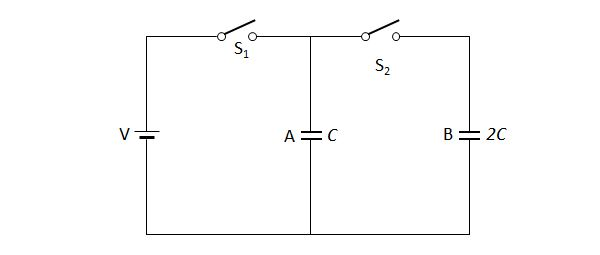# Electric circuitThe above diagram is a circuit that consists of a power supply of voltage $V$ and two capacitors A and B with capacitances $C$ and $2C$, respectively. Suppose that the switch $S_1$ is closed and the switch $S_2$ is open, and sufficient time passes until the quantity of the electric charge on the capacitor A becomes $Q.$ In this state, we open the switch $S_1$ and close the switch $S_2$ and let sufficient time pass. Then what will be the ratio of the quantities of the electric charges on the capacitors A and B, i.e. $Q_A:Q_B?$

×